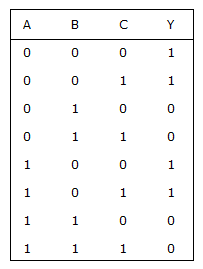# Electronics and Communication Engineering - Digital Electronics - Discussion

27.

For the truth table of the given figure Y =[A]. A + B + C [B]. A + BC [C]. A [D]. B

Explanation:

Y = A B C + A B C + AB C + A B C = A B (C + C) + A B (C + C) = A B + AB = B(A + A) = B.

 Y.Madhu said: (Sep 11, 2016) Explain briefly.

 Gaurang said: (Sep 24, 2016) Draw a 3 variable K-map for output Y and simplify it, you will get an answer.

 Mohammed Umar said: (Dec 13, 2017) I don't get this. Please explain.

 Badhshah said: (Jan 7, 2018) For this, the process is too long. So by substituting those values in options you will get the answer.

 Dhanya said: (Jul 30, 2018) The correct answer is option C.

 Rupinder Kaur said: (Dec 10, 2018) Answer is option D. Please draw k-map of three variables having 8 boxes. AB on one side and C on another side. AB varies 00, 01, 10, 11 and C varies 0, 1. Put values acc to table given of Y=1 in 0, 1, 6 and 7 number box. AB changes from 00 to 10 so discard A because it changes from 0 to 1. Keep B bar because it remains same. In another side C changes from 0 to 1, so discard it also. The answer is bar B because it has no change in it.

 Tshering Dorji said: (Jan 1, 2021) @Rupinder Kaur. Can you work it out in detail?